# Theory of Computation at Columbia

The Theory of Computation group is a part of the Department of Computer Science in the Columbia School of Engineering and Applied Sciences.

We research the fundamental capabilities and limitations of efficient computation. In addition, we use computation as a lens to gain deeper insights into problems from the natural, social, and engineering sciences. Our active research areas include algorithmic game theory, complexity theory, cryptography, the design and analysis of algorithms, interactive computation and communication, theoretical neuroscience, property testing, the role of randomness in computation, sublinear and streaming algorithms, and the theoretical foundations of machine learning.

Our group is highly collaborative, both within Columbia and among peer institutions. We have a weekly Theory Lunch and Student Seminar. We also have an Undergraduate Theory Learning Seminar that organizes student-run reading groups for undergraduates. Most graduate students have (at least) two advisors and collaborate with several professors and other students. Some of our faculty are cross-listed with the IEOR department and the Data Science Institute. We interact with the New York theory community at large through NYCAC, NYC Theory Day, NYC Crypto Day, and the Simons Collaboration on Algorithms and Geometry.

We regularly communicate on two listservs, which you can join on the attached links: theory-read (for seminar talks that include faculty) and theory-phd (for student-centric things, including our student seminar).

Our department and research group are growing, and we're always looking for new members and collaborators. If you would like to join our group as a graduate student, please apply to the PhD program in Computer Science at Columbia. Please reach out to faculty directly for inquiries about postdoc positions.Algorithms, Algebra in Computation, Complexity Theory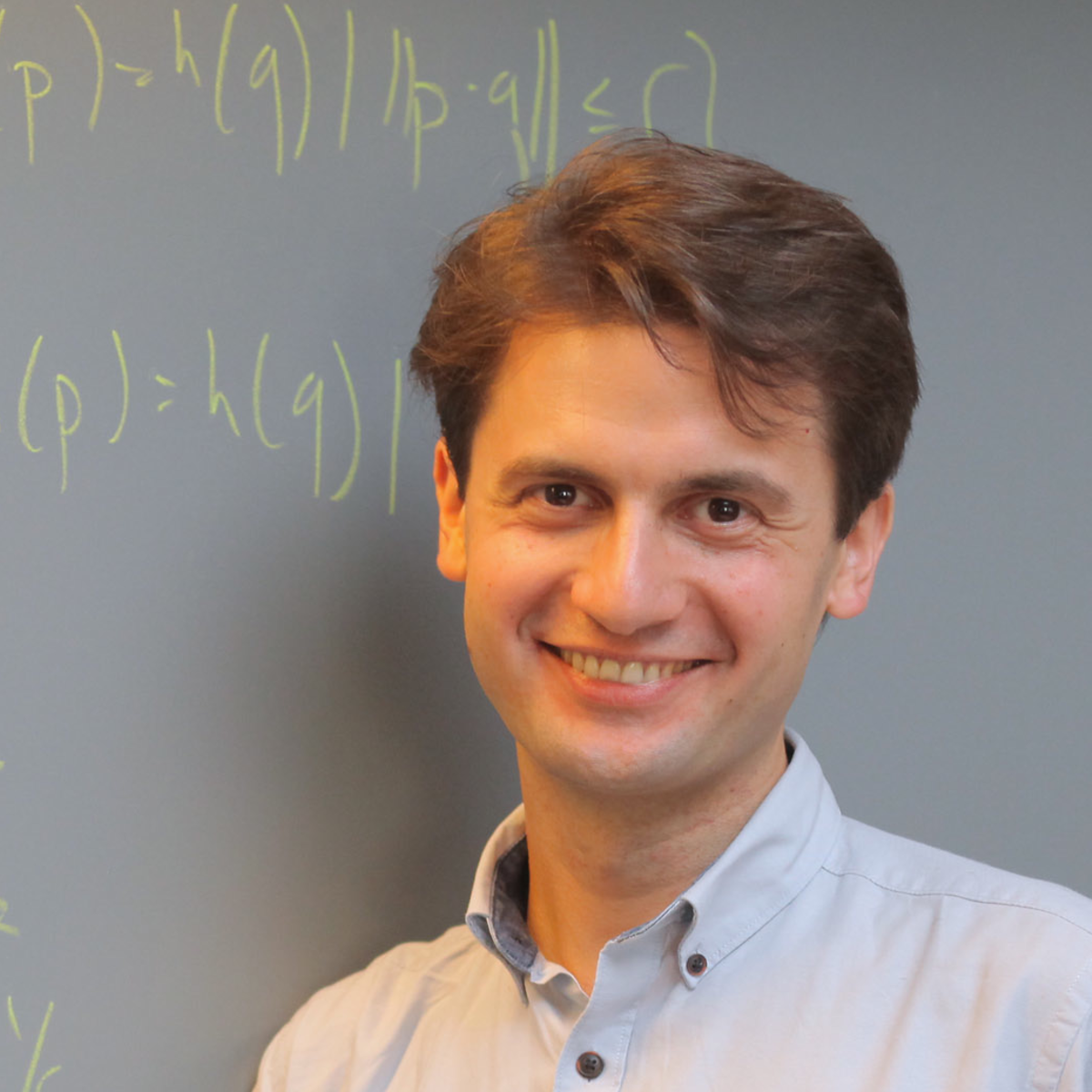Sublinear Algorithms, High-dimensional Geometry, Machine Learning Theory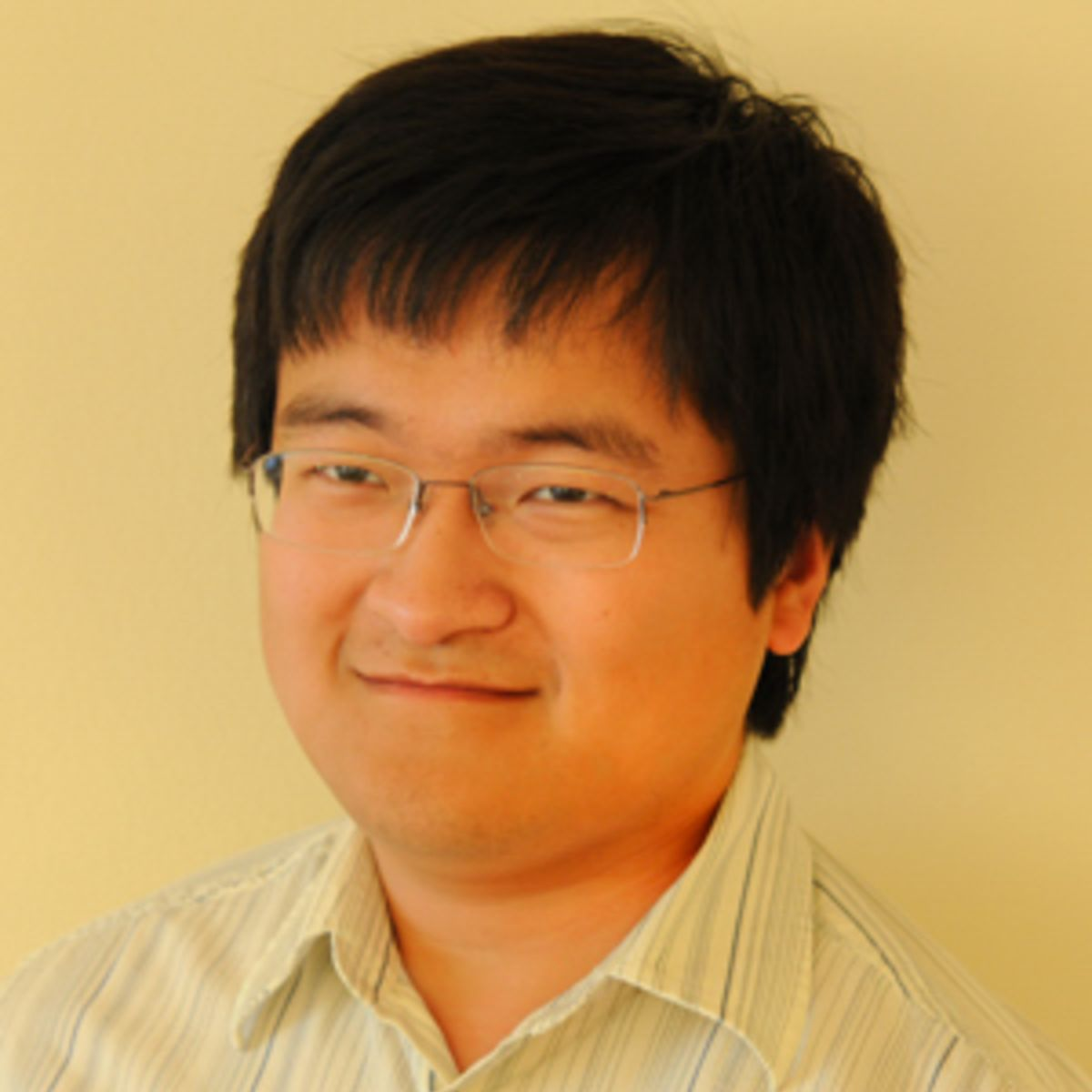Algorithmic Game Theory, Complexity Theory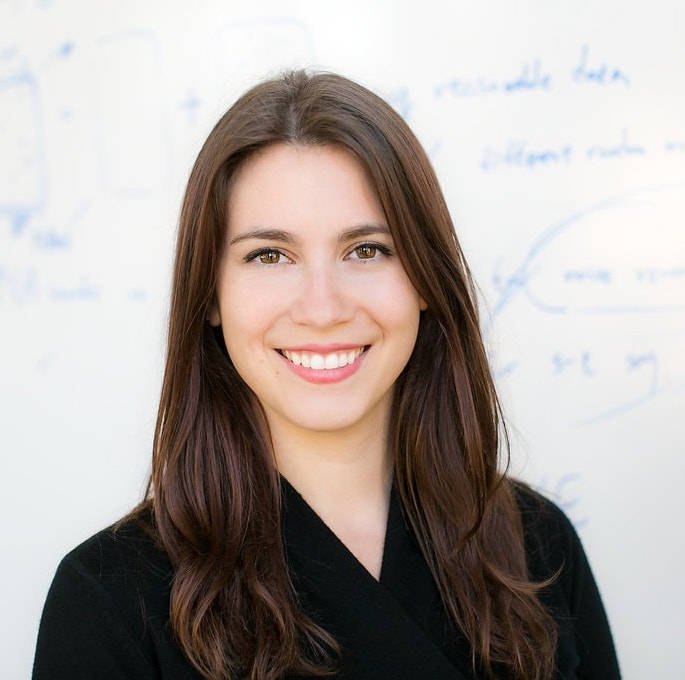Privacy, Algorithmic Game Theory, Machine Learning Theory, Fairness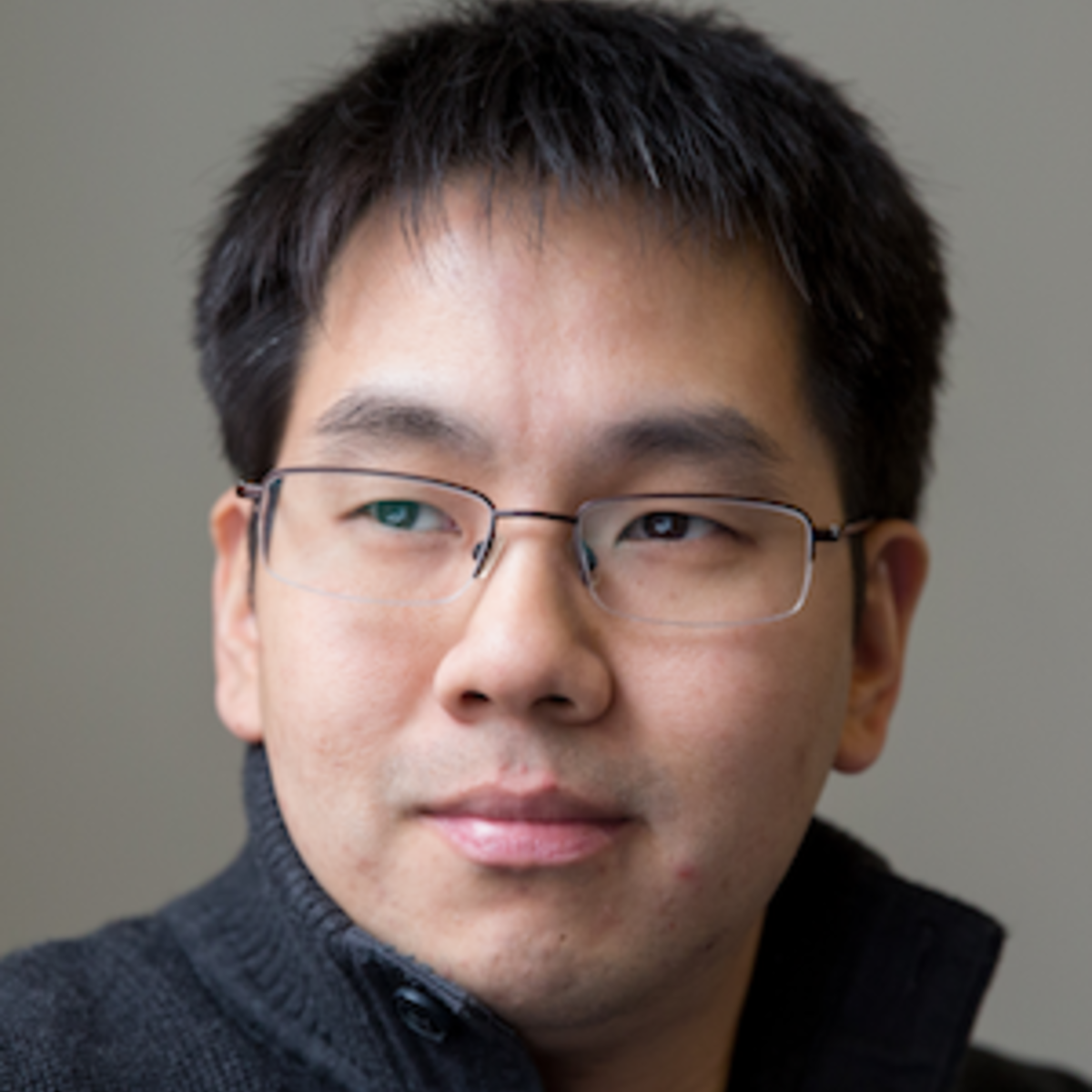Algorithmic Statistics, Machine Learning, Privacy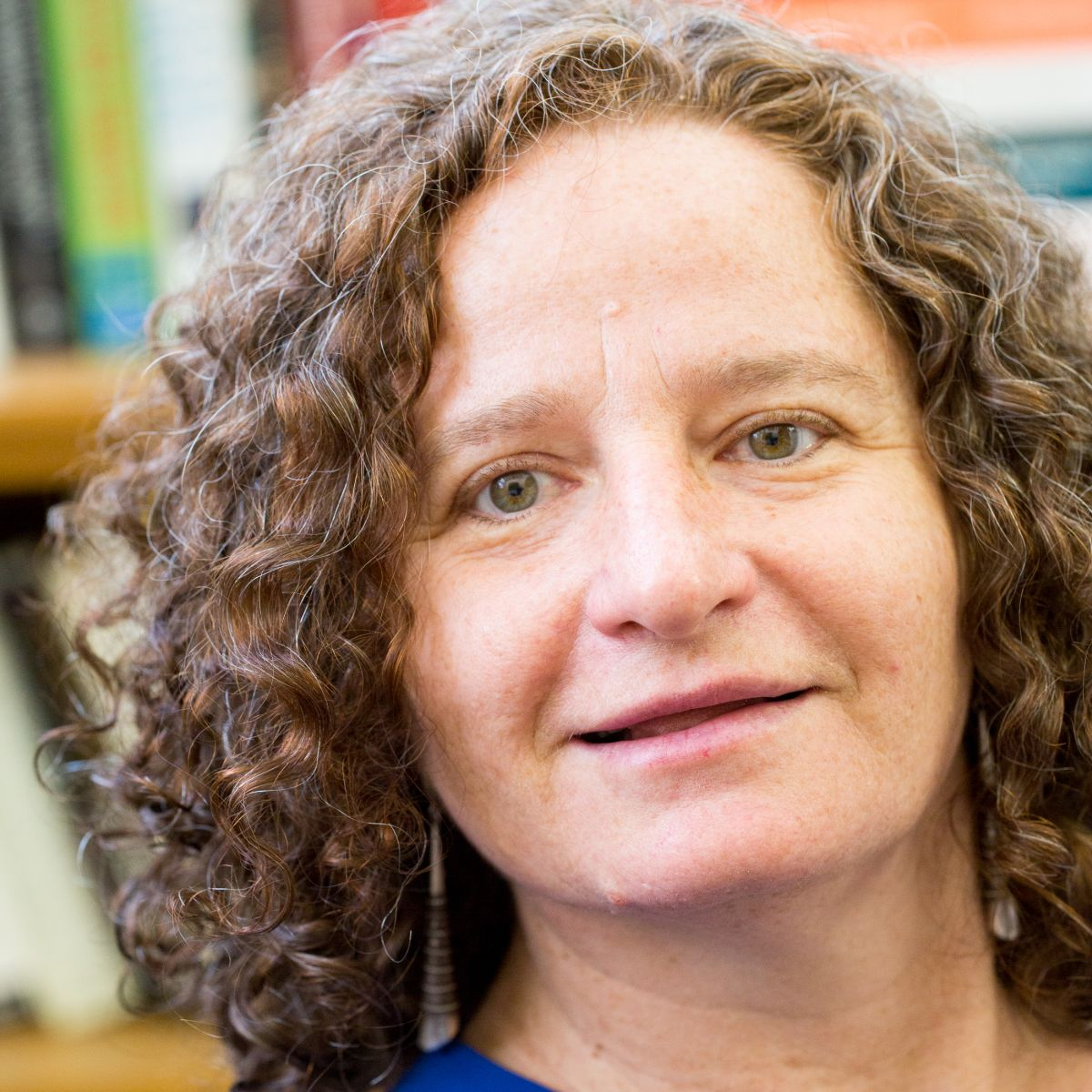Computational Complexity, Cryptography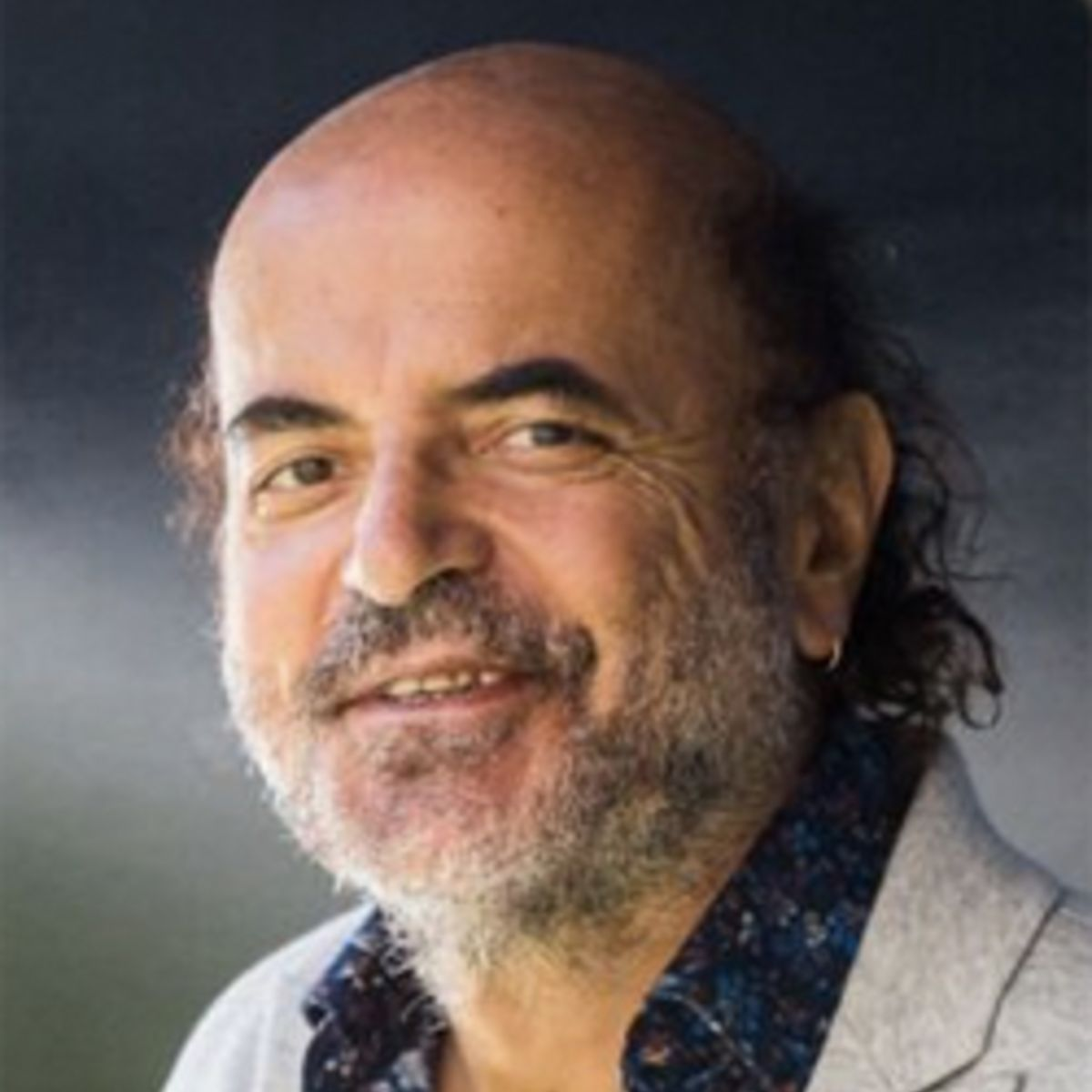Algorithms, Complexity, Algorithmic Game Theory, Evolution, The Brain, LearningComplexity Theory, Communication Complexity, Fairness and Privacy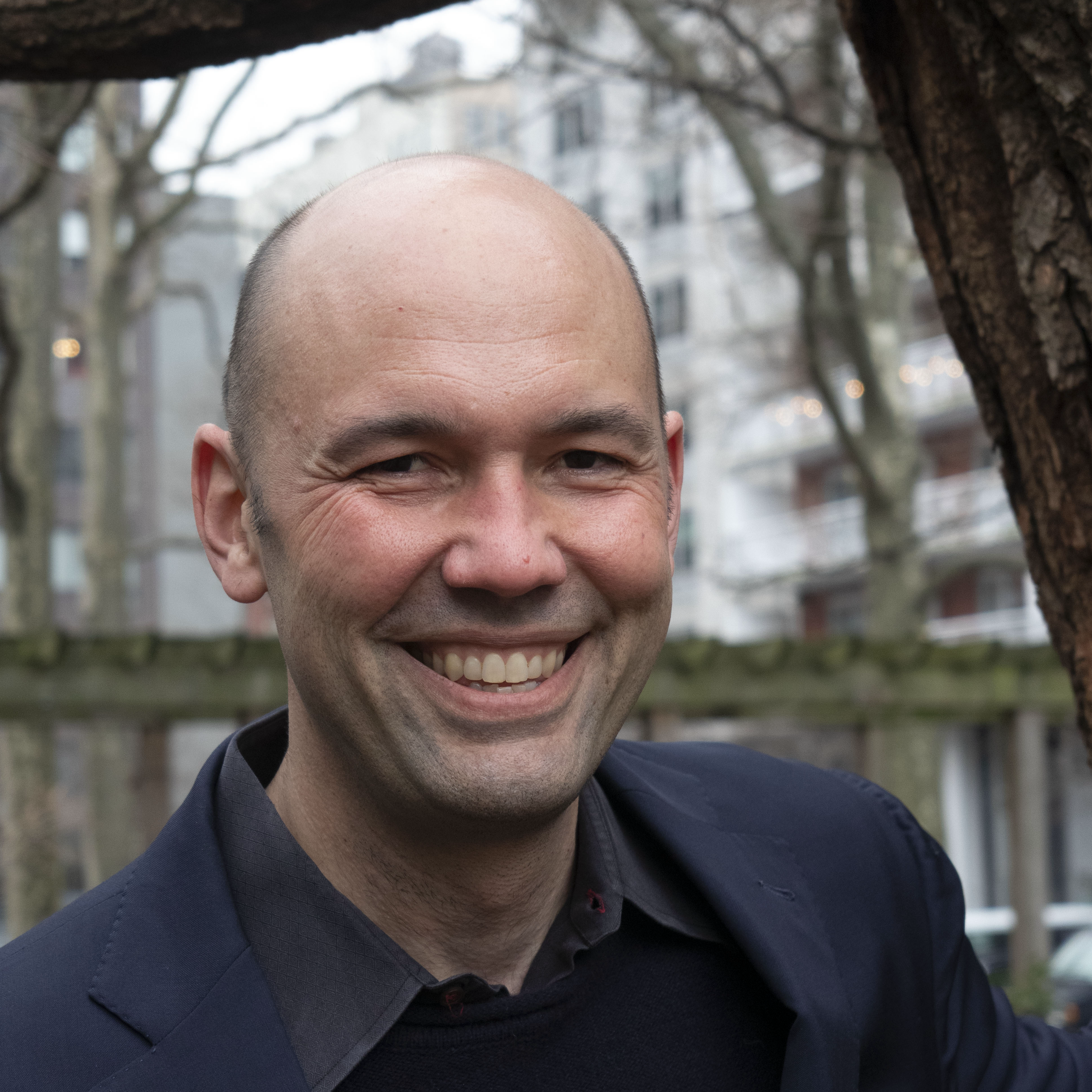Algorithmic Game Theory, Algorithms, Cryptocurrencies, Microeconomics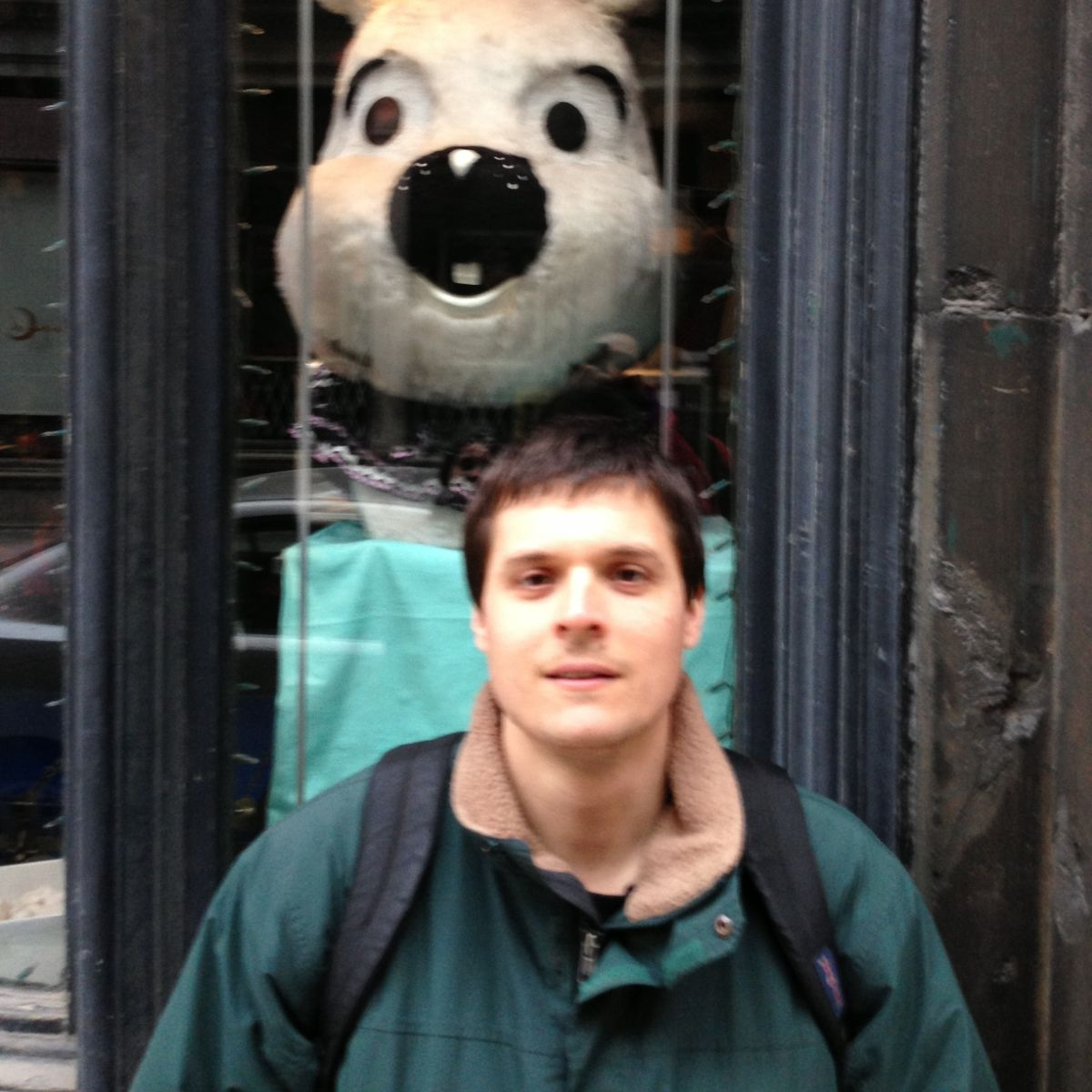Computational Complexity, Learning Theory, Randomness in ComputationAlgorithms, Combinatorial Optimization, Network Algorithms, Scheduling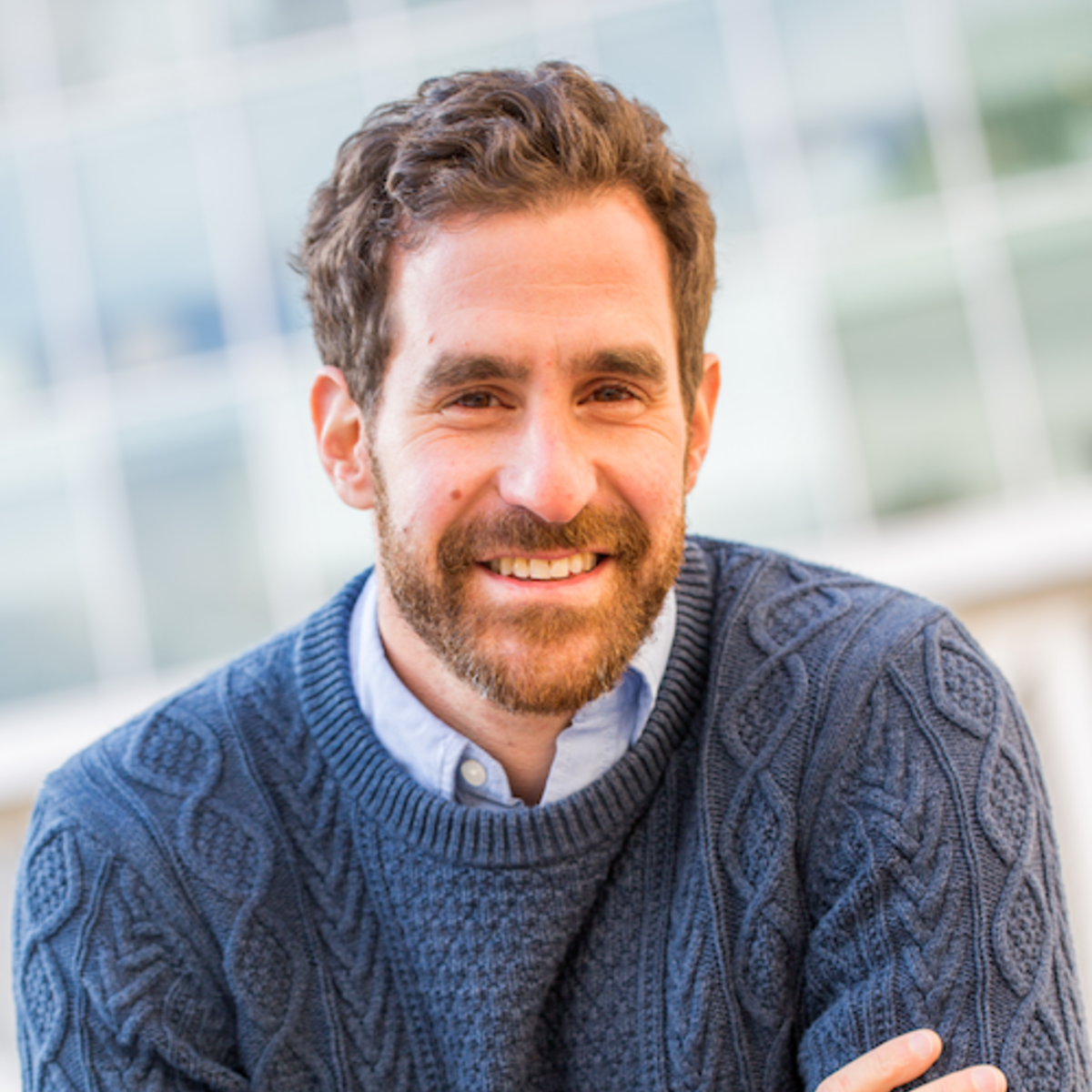Information Theory, Communication Complexity, Data-Structure Lower BoundsAlgorithms, Complexity Theory, Combinatorial Optimization, Databases, Testing and Verification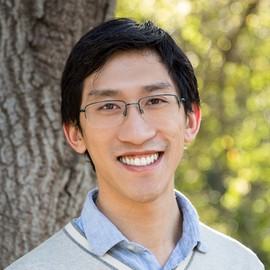Quantum Computing, Complexity Theory, Cryptography, Information Theory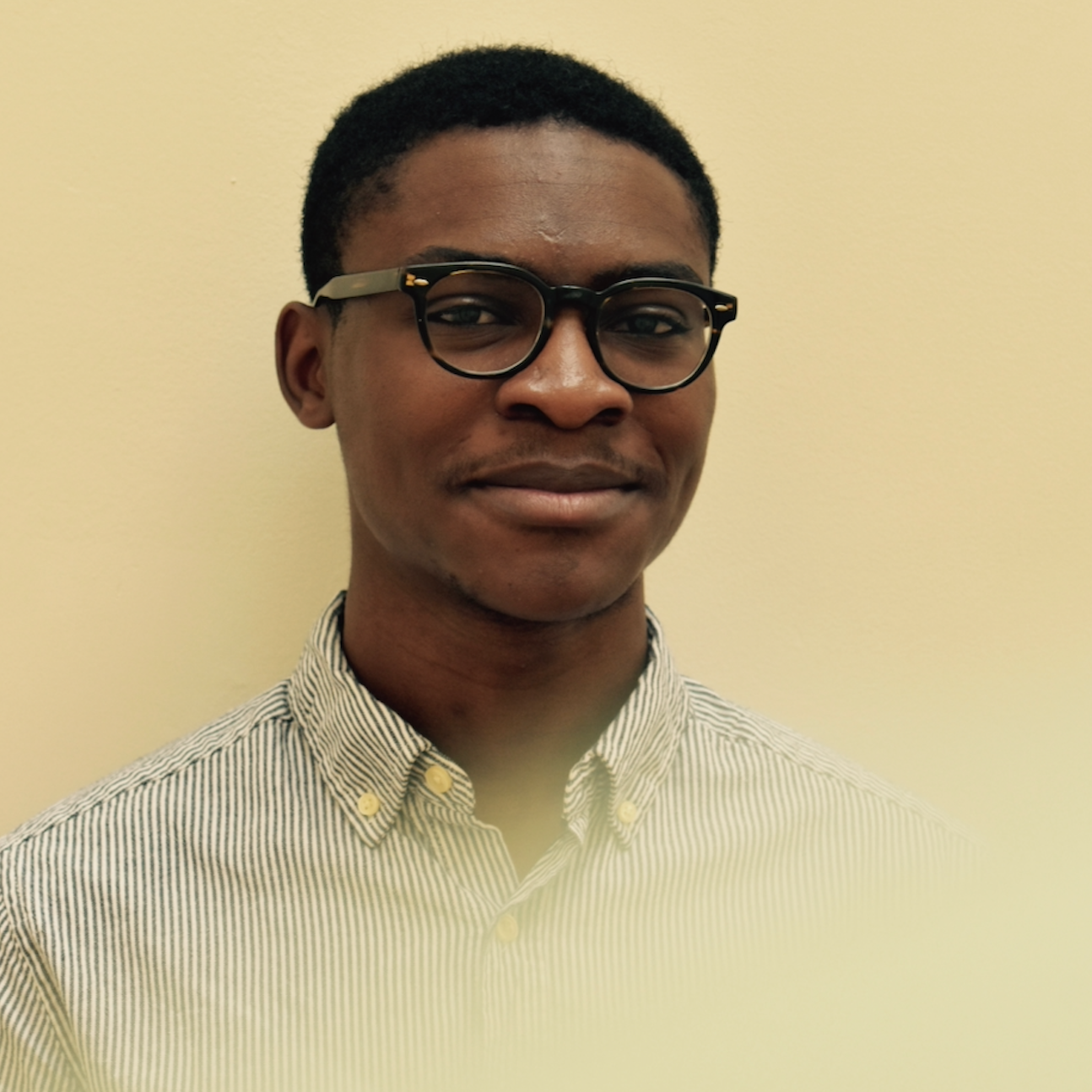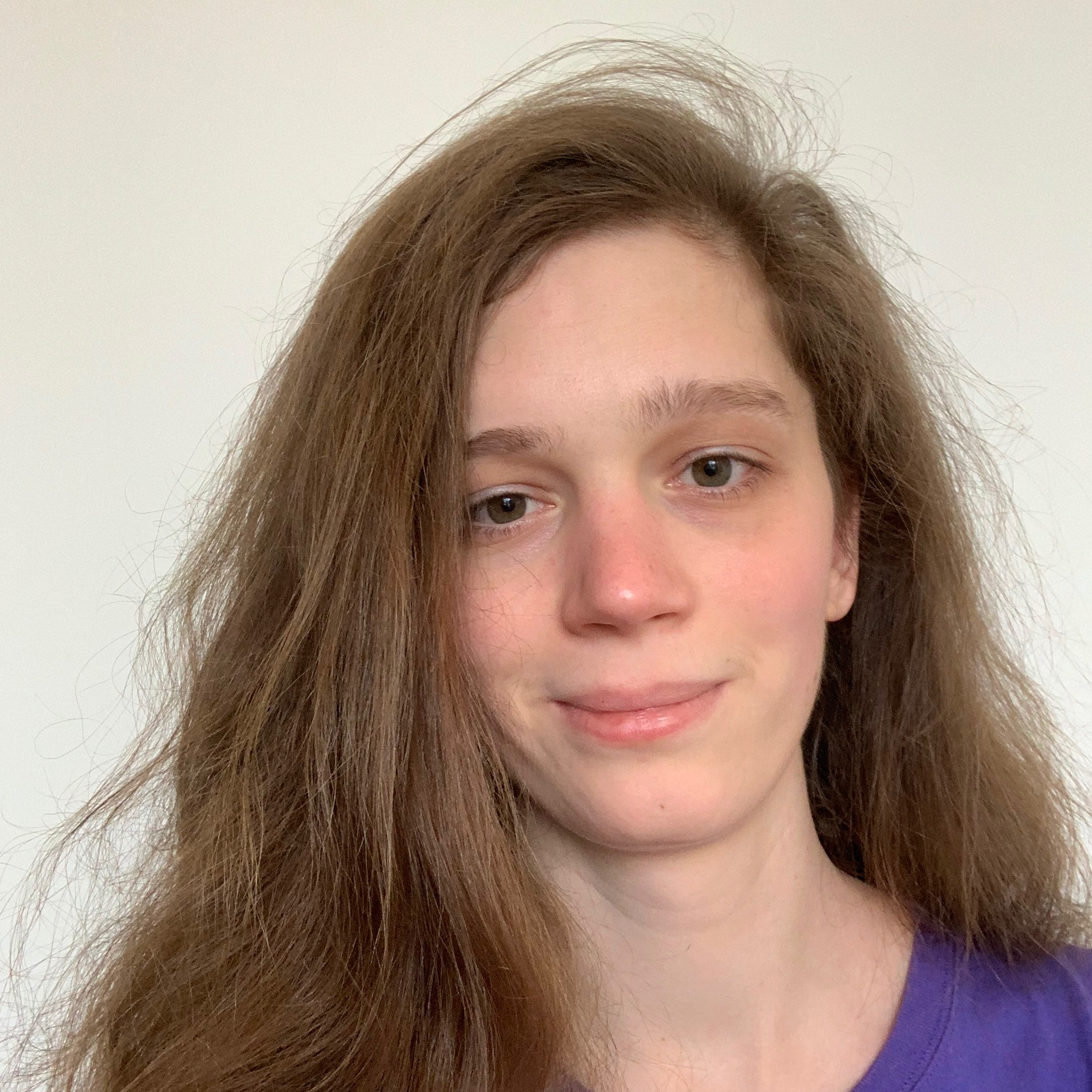Algorithms, Combinatorial OptimizationAlgorithmic Game Theory, Algorithms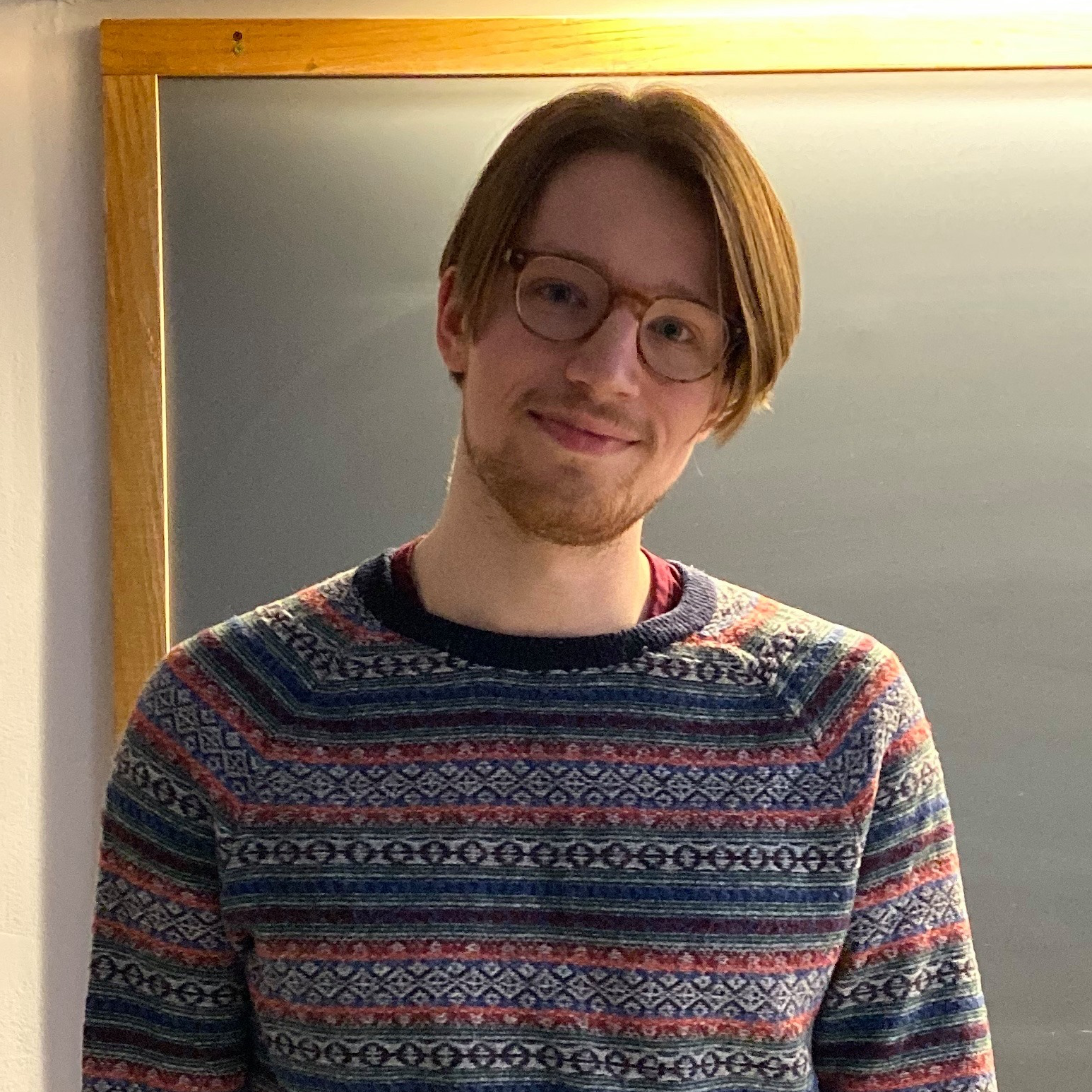Algorithmic Game Theory, Learning Theory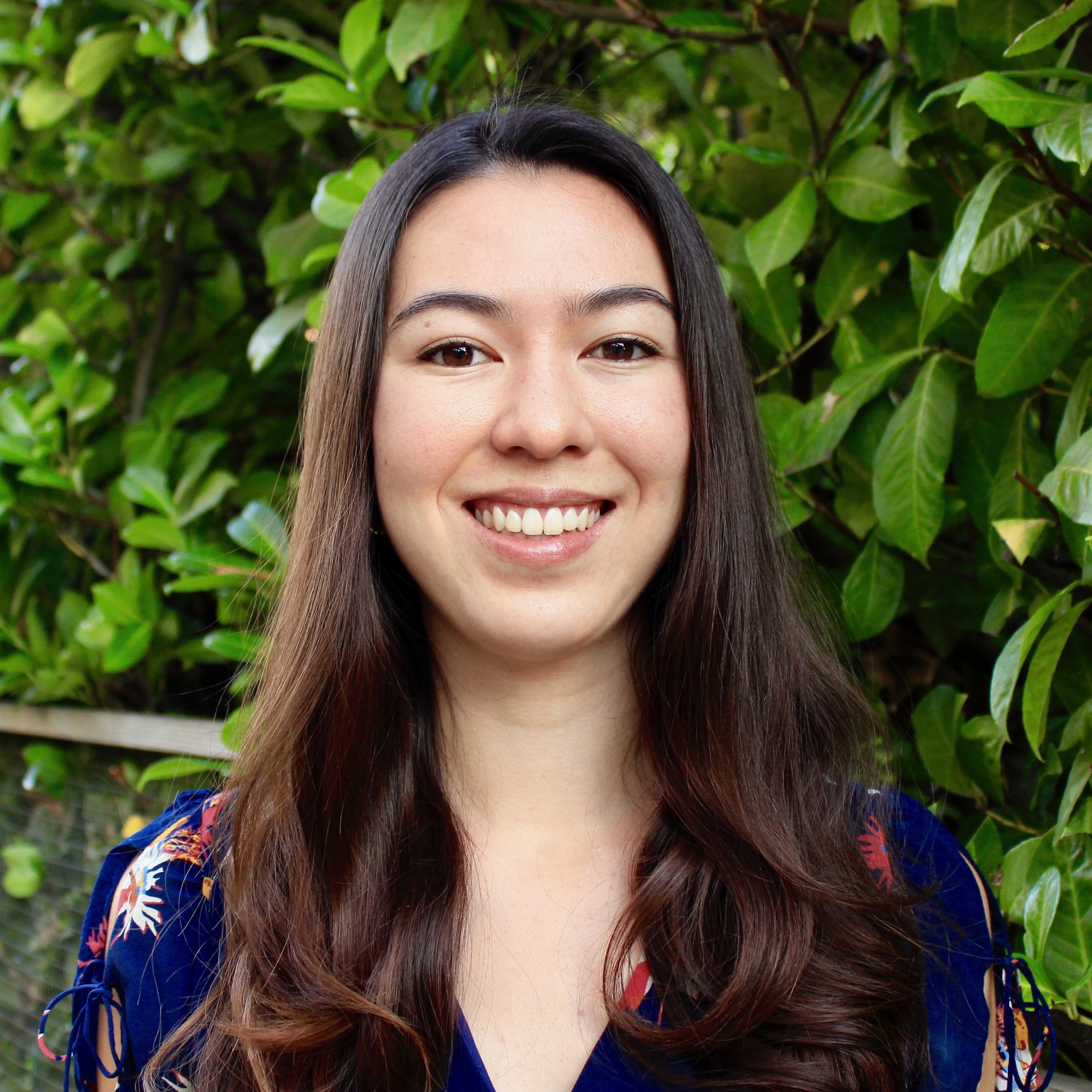Cryptography, Complexity, AlgorithmsSam Deng
Machine Learning Theory, Trustworthy AI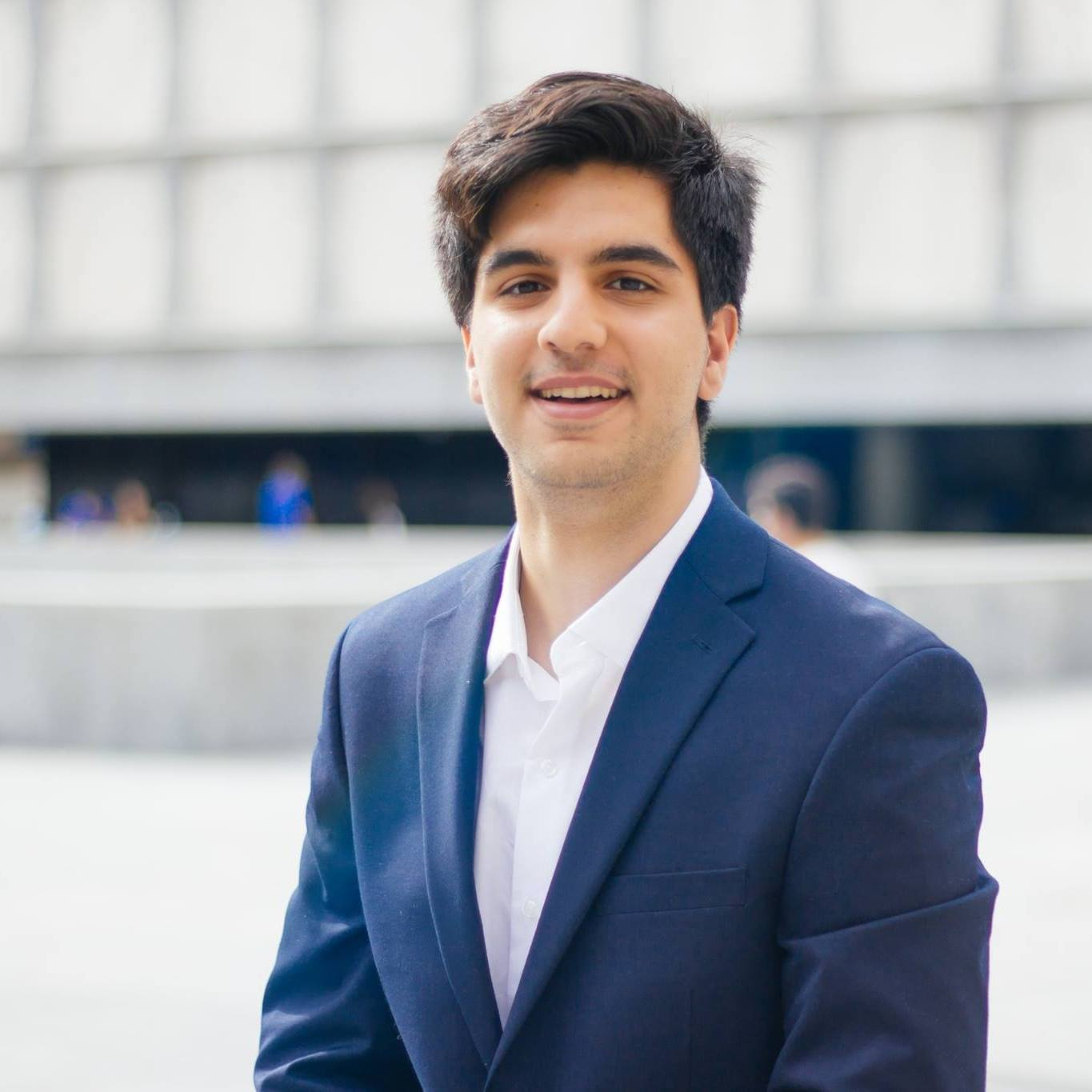Sam Fereidooni
Cognitive Neuroscience, Neurolinguistics, Natural Language ProcessingAlgebraic Techniques, Complexity TheoryData Structures, OptimizationOliver Korten
Complexity Theory, Algorithms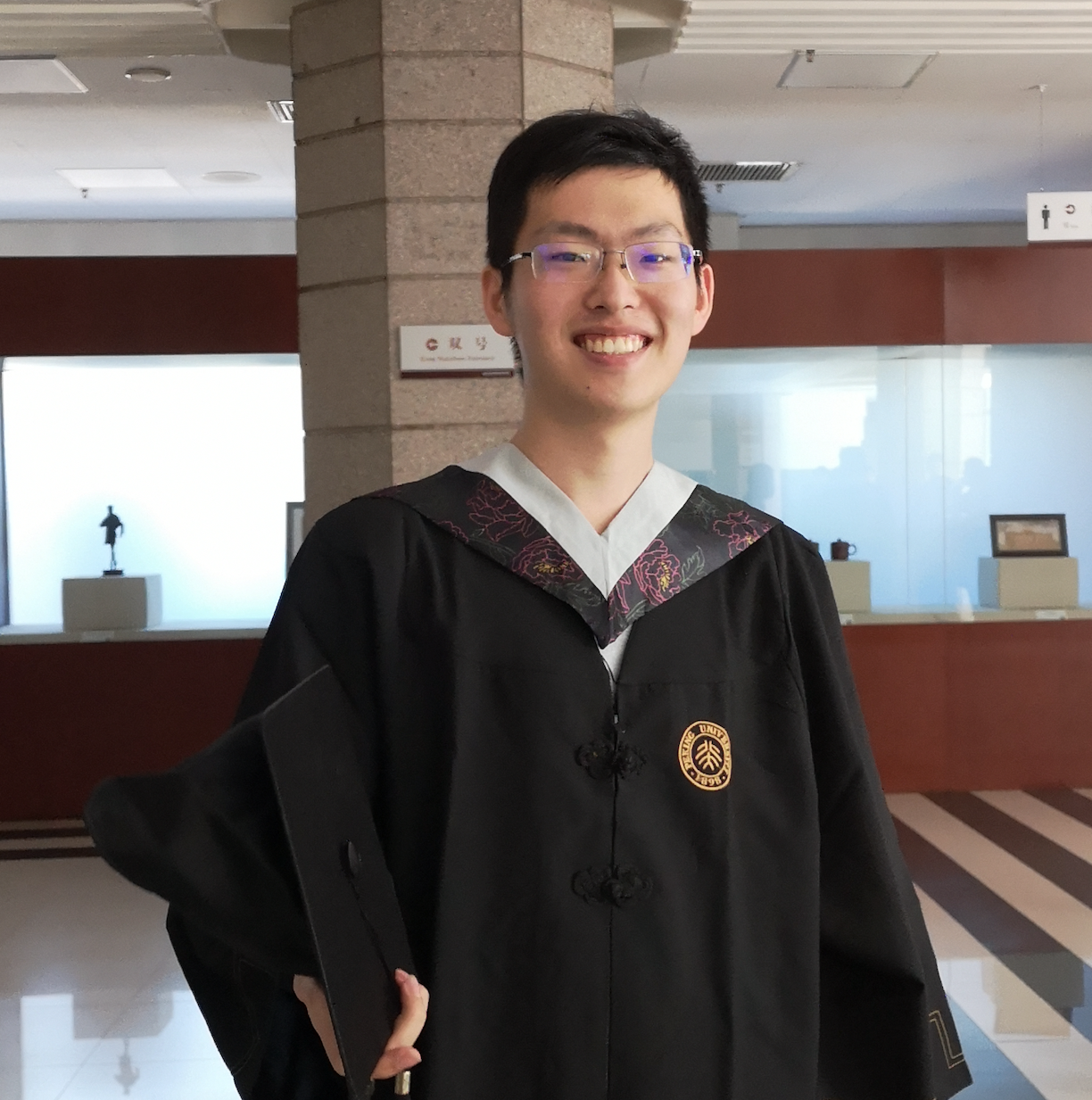Algorithmic Game Theory, Complexity Theory, Learning Theory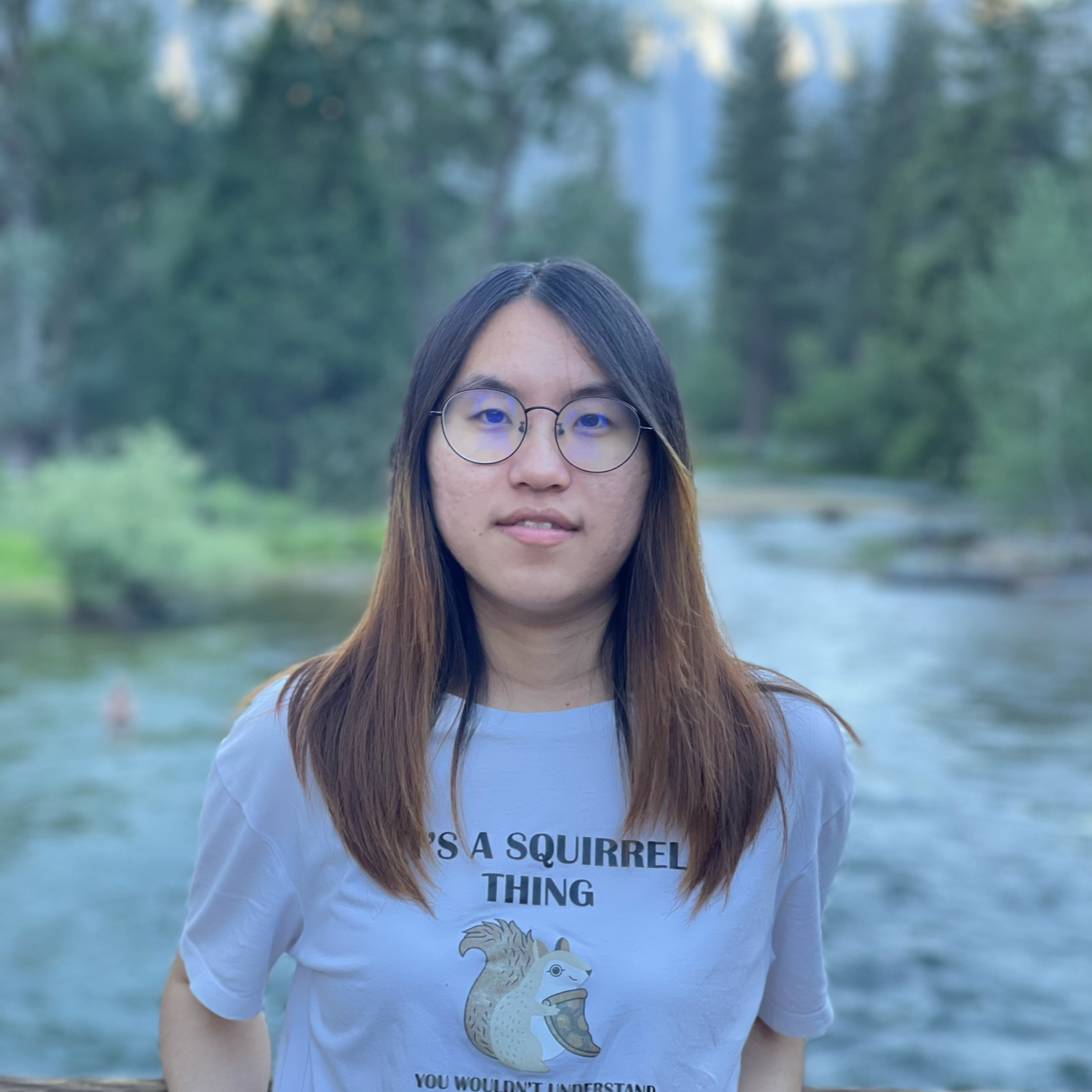Jingwen Liu
Machine Learning Theory, AlgorithmsYasaman Mahdaviyeh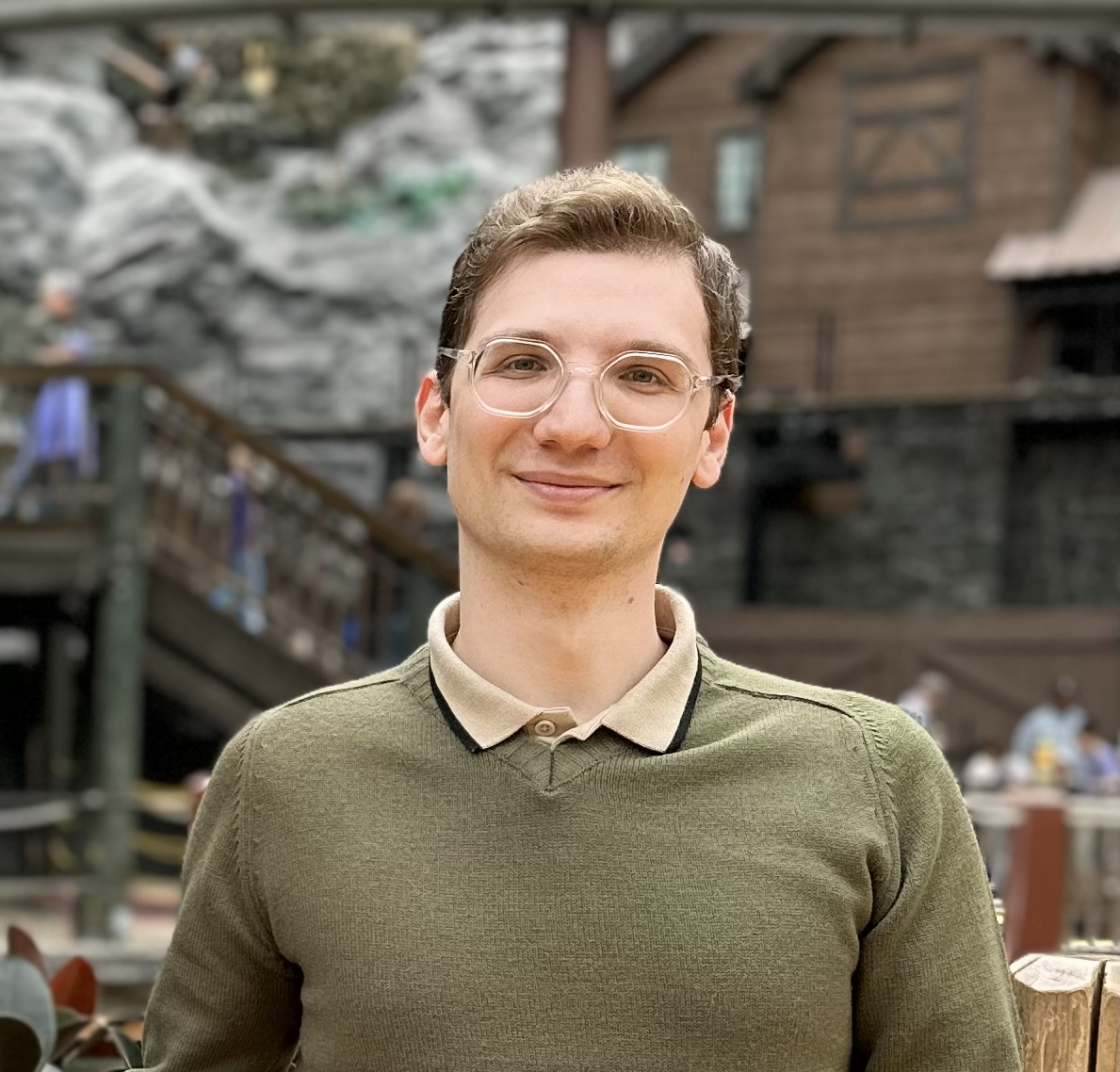Algorithmic Game Theory, Blockchains, ML Theory, ComplexityComplexity, Cryptography, Theoretical Neuroscience, Language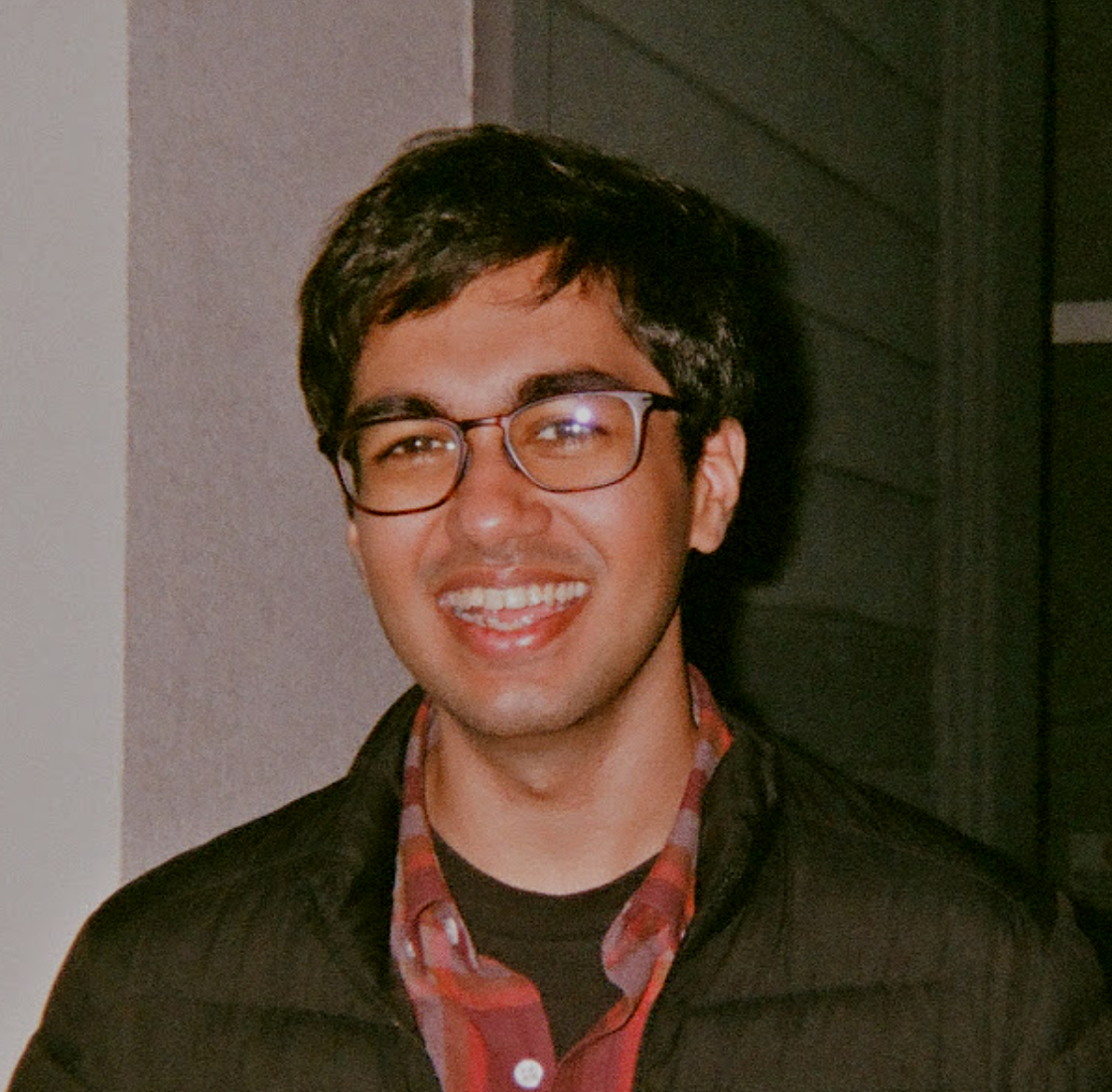Analytic Methods, Complexity Theory, Property TestingAlgorithmic Game Theory, Algorithms, ComplexityQuantum Computing, Complexity Theory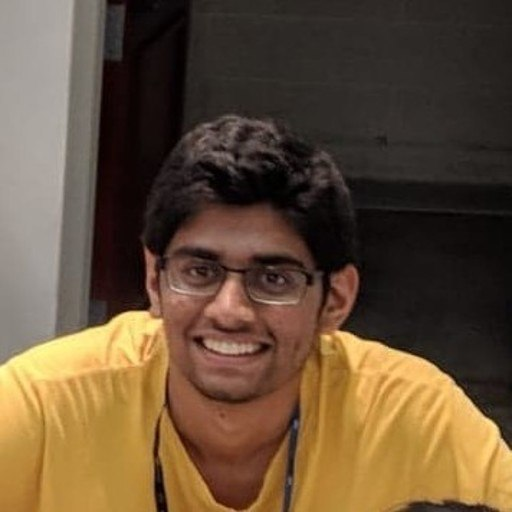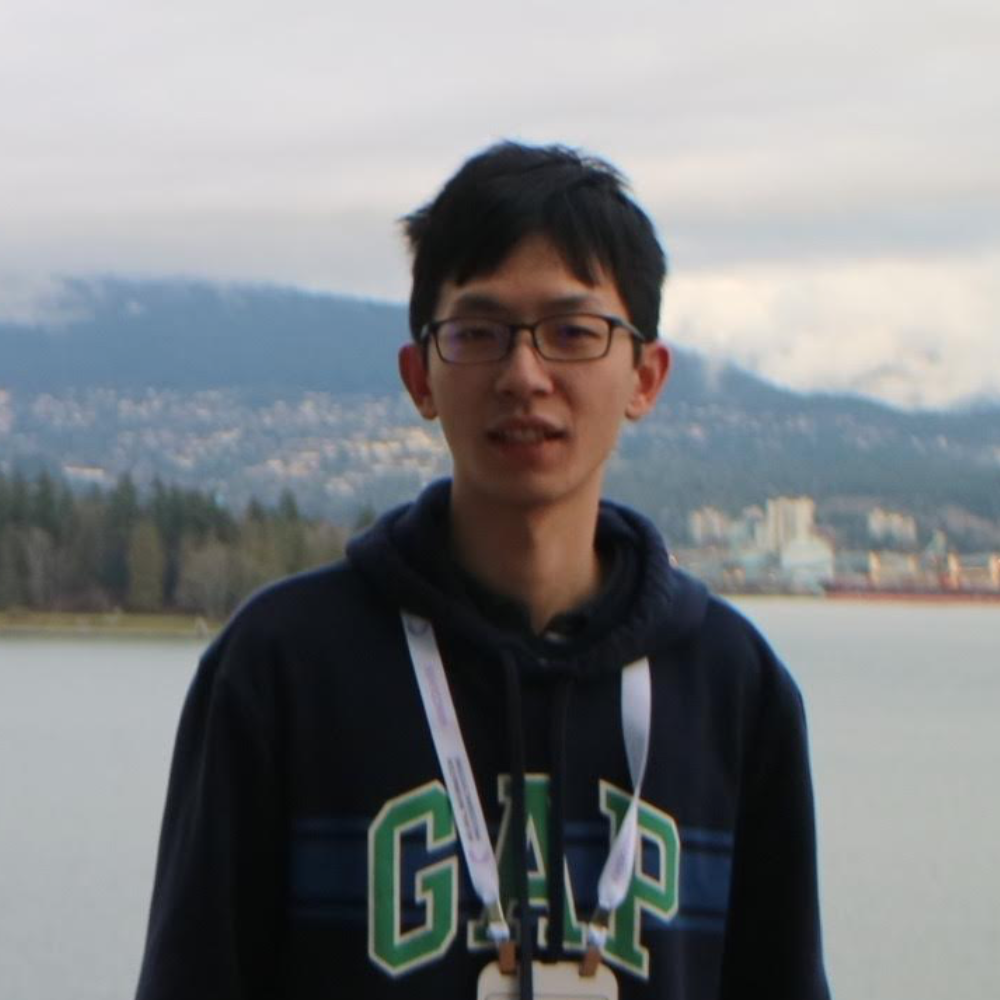Algorithms, Algorithmic Game Theory, Machine Learning, NetworksRandomized Algorithms, Market & Mechanism Design, GraphsAlgorithmic Fairness, Algorithmic Privacy, Causal InferenceMachine Learning Theory, Neural Networks, AI Fairness & AccountabilityNegev Shekel-Nosatzki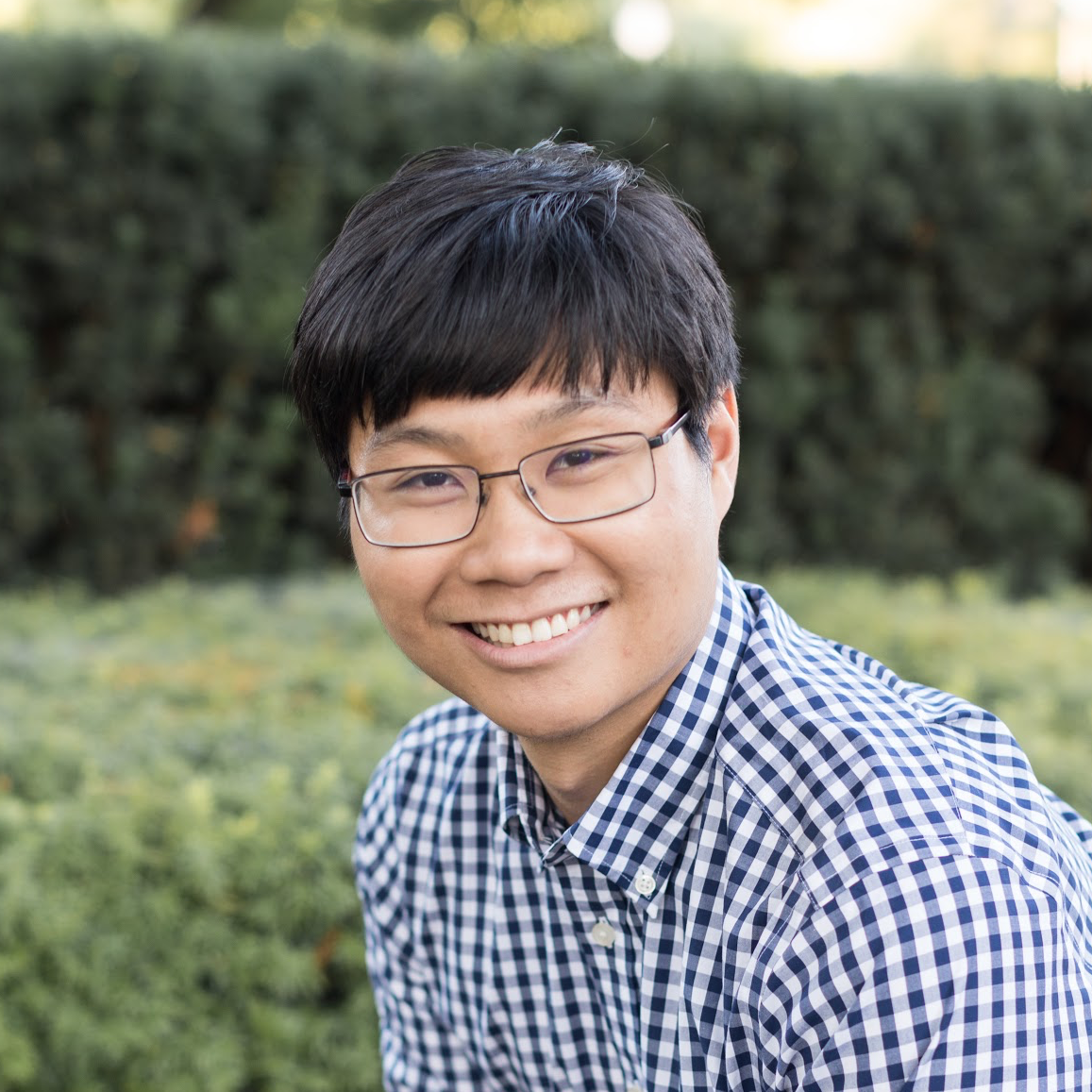Cryptography, Data StructuresAlgorithms, Complexity Theory, Learning Theory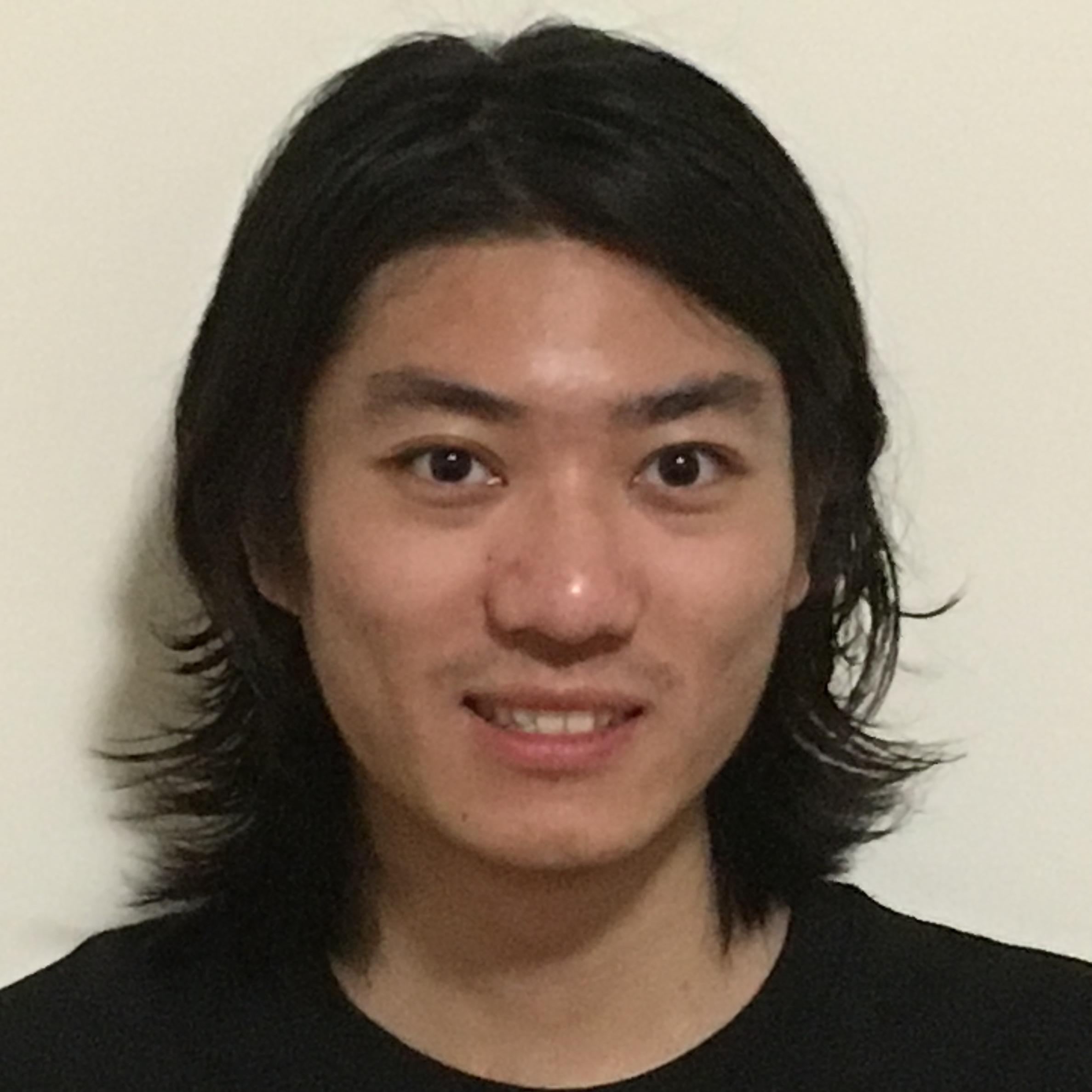Hengjie Zhang
Approximation Algorithms, Data StructuresAlgorithms, Complexity TheoryCryptography, Information Security, Distributed-Ledger Technology